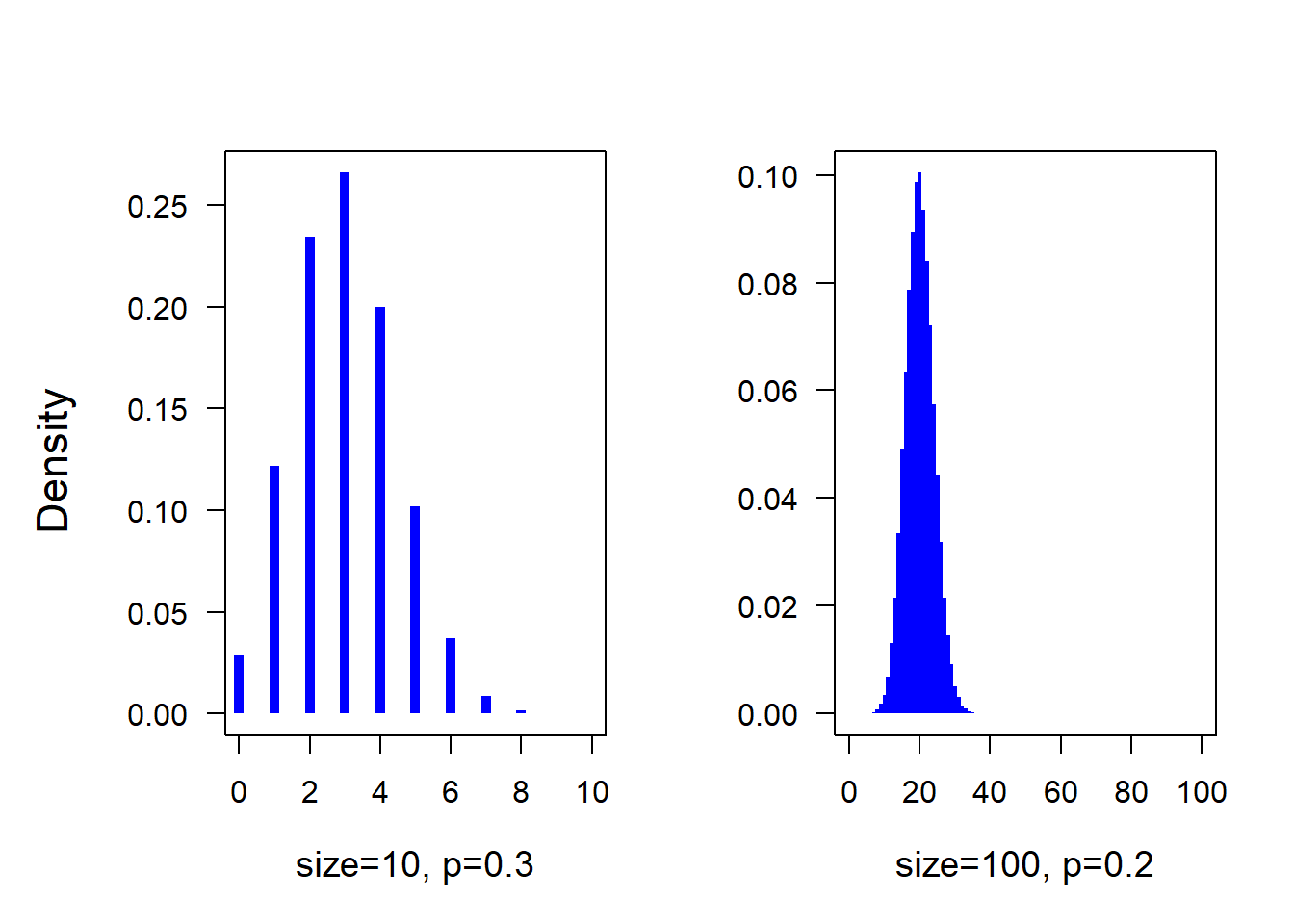# 4 Probability distributions

## 4.2 Normal distribution

normal distribution = Gaussian distribution

$$p(\theta) = \frac{1}{\sqrt{2\pi}\sigma}exp(-\frac{1}{2\sigma^2}(\theta -\mu)^2) = Normal(\mu, \sigma)$$

$$E(\theta) = \mu$$, $$var(\theta) = \sigma^2$$, $$mode(\theta) = \mu$$

The variance parameter can be specified to be a variance, a standard deviation or a precision. Depending on the software used (or author of the paper) different habits exist.

xxx

## 4.4 Gamma distribution

xxx

### 4.4.1 Cauchy distribution

We sometimes use the Cauchy distiribution to specify the prior distirbution of the standart deviation and similar parameters in a model. An example for this is the regression model that we used to introduce Stan (Chapter 18.3).

### 4.4.2 t-distribution

marginal posterior distribution of a normal mean with unknown variance and conjugate prior distribution

$$p(\theta|v,\mu,\sigma) = \frac{\Gamma((v+1)/2)}{\Gamma(v/2)\sqrt{v\pi}\sigma}(1+\frac{1}{v}(\frac{\theta-\mu}{\sigma})^2)^{-(v+1)/2}$$

$$v$$ degrees of freedom
$$\mu$$ location
$$\sigma$$ scale

## 4.5 F-distribution

Ratios of sample variances drawn from populations with equal variances follow an F-distribution. The density function of the F-distribution is even more complicated than the one of the t-distribution! We do not copy it here. Further, we have not yet met any Bayesian example where the F-distribution is used (that does not mean that there is no). It is used in frequentist analyses in order to compare variances, and, within the ANOVA, to compare means between groups. If two variances only differ because of natural variance in the data (nullhypothesis) then $$\frac{Var(X_1)}{Var(X_2)}\sim F_{df_1,df_2}$$.Figure 4.1: Different density functions of the F statistics